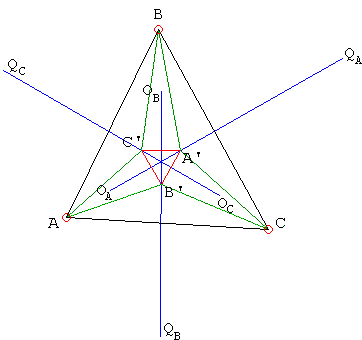### This applet requires Sun's Java VM 2 which your browser may perceive as a popup. Which it is not. If you want to see the applet work, visit Sun's website at https://www.java.com/en/download/index.jsp, download and install Java VM and enjoy the applet.

 What if applet does not run?

(The numbers are clickable and so may be modified.)What's happening is this. Let r be a number between 0 and 1. We shall perform a construction similar to but more general than Morley's. For vertex A, measure angles rA from both sides, and similarly for the vertices B and C. The lines adjacent to one of the sides of the given triangle intersect forming seven triangles: the middle one -- Morley's if r = 1/3 -- and 3 pairs of "opposite" triangles. It appears that the lines joining incenters of the opposite triangles are concurrent. It also seems to be true of the circumcenters. We can prove the latter when r = 1/3.### Theorem (by Nikos Dergiades with an important correction by Samuele Mongodi)

Let A'B'C' denote the Morley triangle. Denote the circumcenters of triangles AB'C', BC'A', and CA'B' OA, OB and OC, and those of triangles BCA', CAB', and ABC' QA, QB and QC, respectively. Let angles A = 3x, B = 3y, C = 3z.

Since it's known that ∠BA'C' = 60° + z, ∠BQAA' = 2z (as central angle subtending the same arc as the inscribed angle BCA') and ∠QAA'B = 90° - z (see below) we have ∠QAA'C' = 150°, which means that the line QAA' is the bisector of angle A' in Morley's triangle A'B'C'. Hence, it's also the perpendicular bisector of B'C'. As such, it passes through OA.

Similar statements are true with regard to OBQB and OCQC, which means that the three lines meet at the center of Morley's triangle.

(Some of the triangles have already been considered elsewhere.)Samuele Mongodi found a flaw in the original Nikos' argument and offered the following fix:

Since QA is the circumcenter of ΔBCA', ΔBA'QA is isosceles, so that ∠QABA' = ∠QAA'B. In combination with ∠BQAA' = 2z, as noted by Nikos, this yields

 ∠QAA'B = (1800 - 2z)/2 = 900 - z,

as claimed.